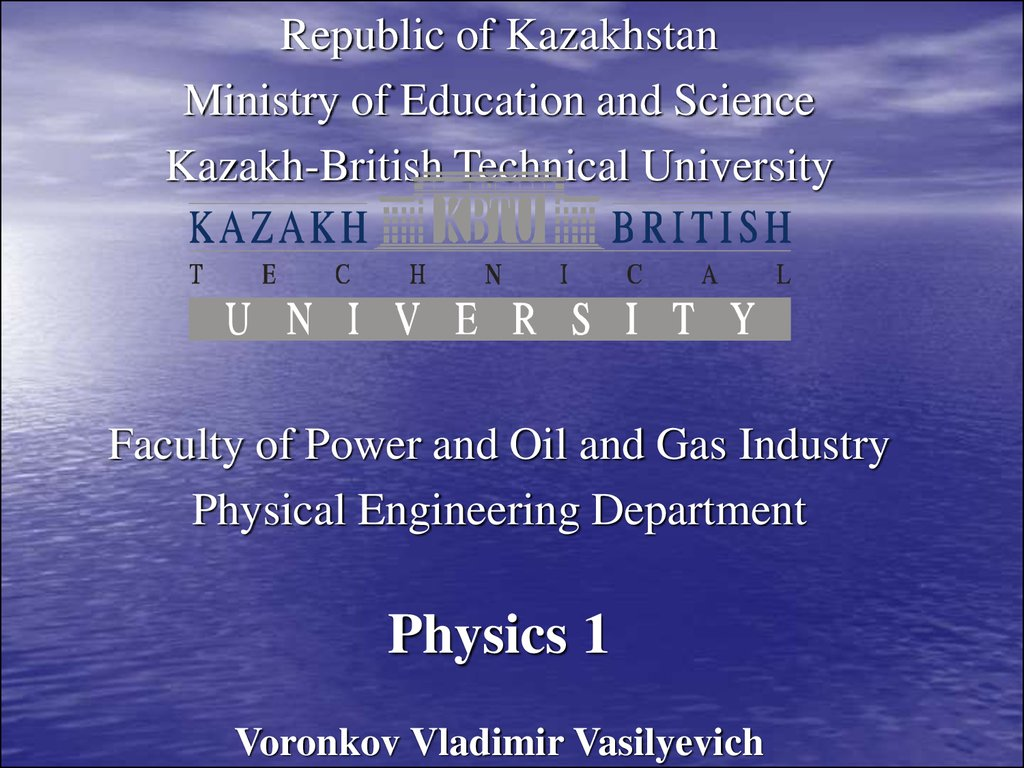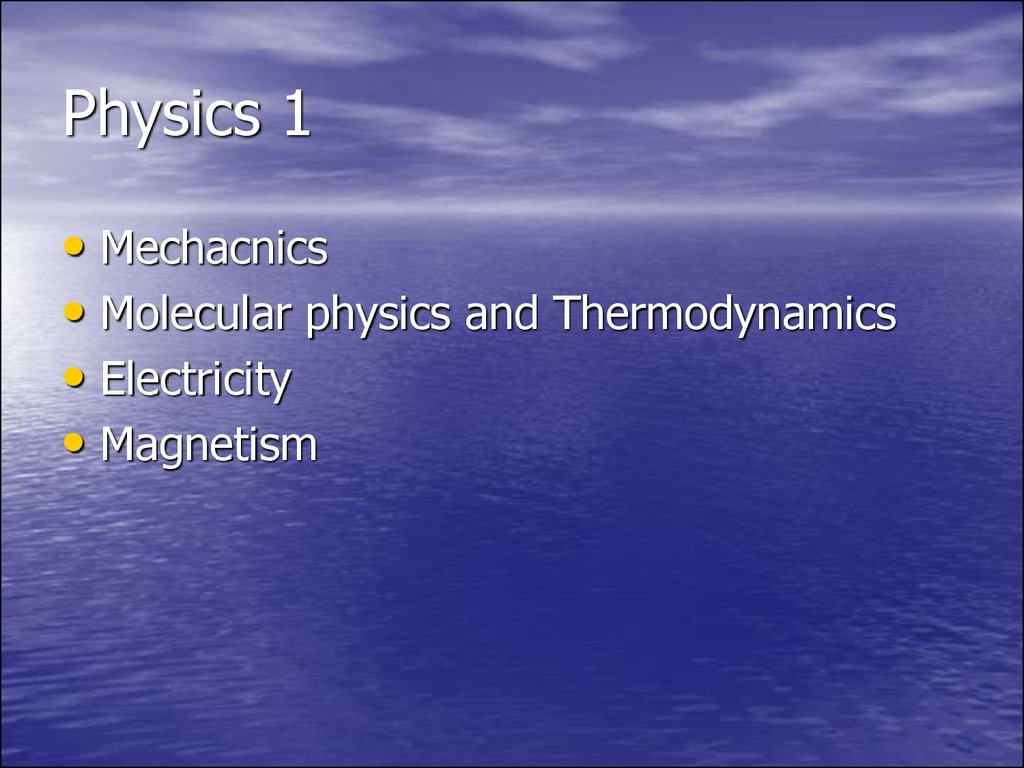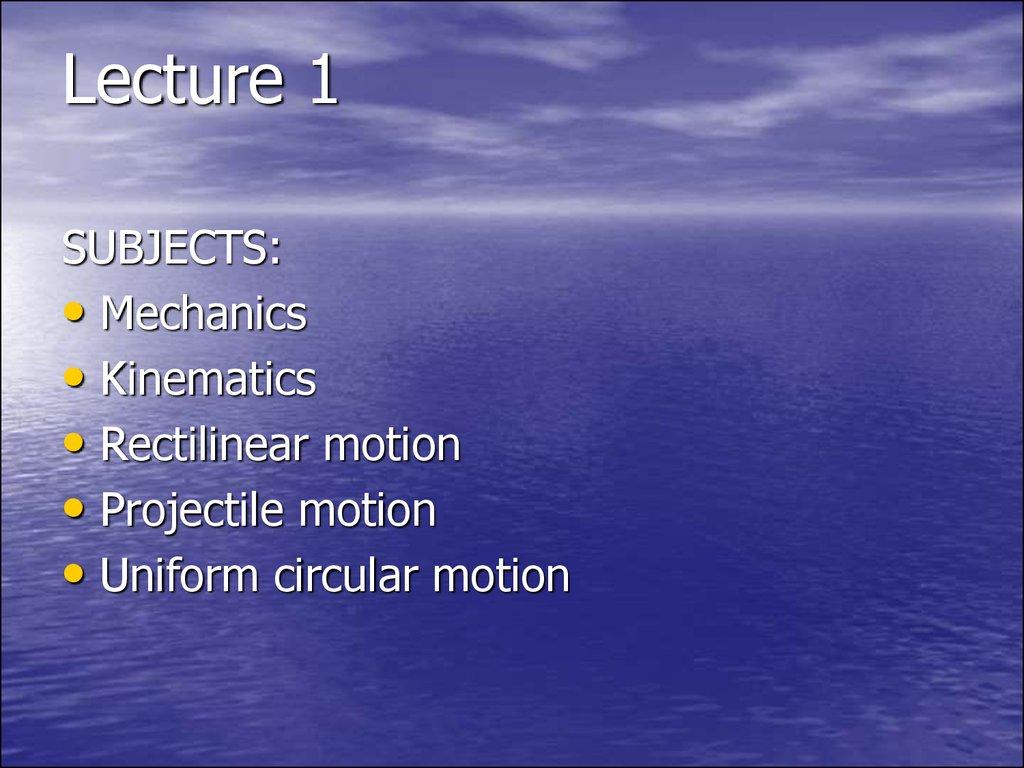# Mechacnics. Molecular physics and Thermodynamics. Electricity. Magnetism

## 1.

Republic of Kazakhstan
Ministry of Education and Science
Kazakh-British Technical University
Faculty of Power and Oil and Gas Industry
Physical Engineering Department
Physics 1

## 2. Physics 1

• Mechacnics
• Molecular physics and Thermodynamics
• Electricity
• Magnetism

## 3. Lecture 1

SUBJECTS:
• Mechanics
• Kinematics
• Rectilinear motion
• Projectile motion
• Uniform circular motion

## 4. Mechanics

• Mechanics is the science of motion and its
cause.
• Kinematics is the mathematical description
of motion.

## 5. Main terms of Kinematics

• Displacement is the change in the position of an object.
one-dimensional:
∆x= x2 - x1
many-dimensional: x x1 x2
Average velocity is the distance traveled per unit of
time:
x
v
t
• Instantaneous velocity is the velocity at infinitely
small interval:
x
v lim
t 0 t
or the same
dx
v
dt

## 6.

• Average acceleration is the total change in
velocity per unit of time:
v
a
t
• Instantaneous acceleration is the change in
velocity per unit of time at infinitely small time:
dv
a
dt
• Gravitational motion is the motion when
gravitational acceleration g=9.8 m/s2 takes
part. For example: rocket motion.

## 7.

• Displacement at constant acceleration in
rectilinear motion :
2
at
r r0 V0t
2
• Where r0 and V0 is initial displacement and
velocity at t=0, a is constant acceleration.

## 8. Rectilinear motion in graphics

A) Object stands still.
B) Object moves with constant speed.

## 10.

• The car motion in table

dx
v
dt

x
v lim
t 0 t

dv
a
dt

## 16.

• Projectile motion is a gravitational
motion but the object has no its own
acceleration. So the motion goes with
constant gravitational acceleration. For
example: cannonball motion.
• Usual method for solving projectile motion
problems:
– Separate the motion into two parts: vertical
and horizontal: so we have:
• two coordinates x and y
• two velocities Vx and Vy
• one acceleration ay =-g, and ax=0

## 17.

– Then one should determine the elevation angle Θ0 the initial angle to horizon.
– So the trajectory of an object in the gravitational field
can be described as following:
x= V0CosΘ0 t,
y= V0SinΘ0 t – gt2/2.
Let’s designate R as the range the object travels from
zero height (y=0) till its fall (y=0 again) then we can
calculate it as
R=V02Sin(2Θ0)/g.
Flight time t: it’s easy (using the equation dy/dt = 0) to
find that the time of ascent is V0SinΘ0/g, then the full
flight time is double:
tflight= 2V0SinΘ0/g.
Using the flight time one can find:
– the maximal height,
– the range of flight (the maximum range of flight from
zero height (y=0) till the fall of the object (y=0
again)).

R =V02Sin(2Θ0)/g

## 20. Circular uniform motion

• Uniform circular motion is performed with
constant speed along a circular path. Circular
motion is a special case of motion on a plane. Its
coordinates is angular coordinate φ and radius r.
The angular speed w is defined as:
d
w
dt
• The linear speed relates to the angular speed as:
v wr
• Period T is the time of one full revolution:
T= 2p/w.

• Displacement
• Velocity
• Acceleration
• Angle
• Angular speed
• Period
x,y
V
a,g
φ
w
T
m
m/s
m/s2